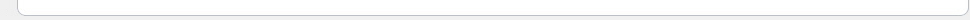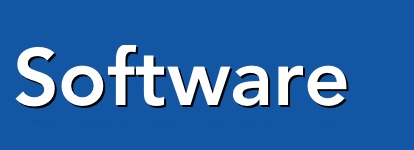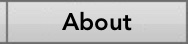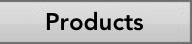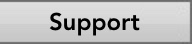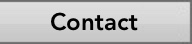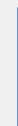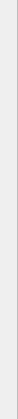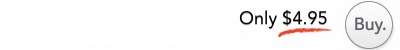Attention students and teachers! Announcing the all new Trigonometry calculation software from Praeter Software, TRIGAID is the tool that you need to calculate Trig formulas more quickly and accurately than ever before!

The perfect companion for when you're studying or doing homework, TrigAid helps you learn the material by showing you the formula and the correct calculation, AND it helps you save time on your assignments by giving you the correct calculation with just a few keystrokes and a click of the mouse! With TrigAid, you can say goodbye to the long hours of searching for the correct formula and tedious repetition, and the frustration of not knowing if did the calculations correctly until the professor hands back your assignment.

TrigAid has the following features: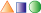Geometry - Calculate every variable in eight different geometric shapes, including cones, spheres, and cylinders!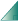Triangulator - Calculate the angles, area, and side lengths of any triangle!Trig Laws - Law of Sines, Cosines, Pythagorus' Theorem, and ofcourse SOHCAHTOA!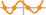Zeroes - Quickly find the x-intercepts of a polynomialLogarithms -- Work with relationship of logarithms and exponents and moreVectors - Calculate the magnitude and angles of up to eight vectors!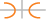Conics -- Calculate the ellipse, parabola, and hyperbola's center, foci and vertexes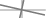Axes Rotation -- Eliminate the xy term of the polynomial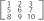Matrices - Add, subtract, and multiply matricies up to 5x5!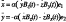Linear Equations - Solve for up to five unknowns!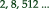Sequences - Find any term in arithmetic AND geometric sequences!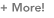Additionally - Quadratic Formula, unit converter, quick calculator and a polar to rectangular converter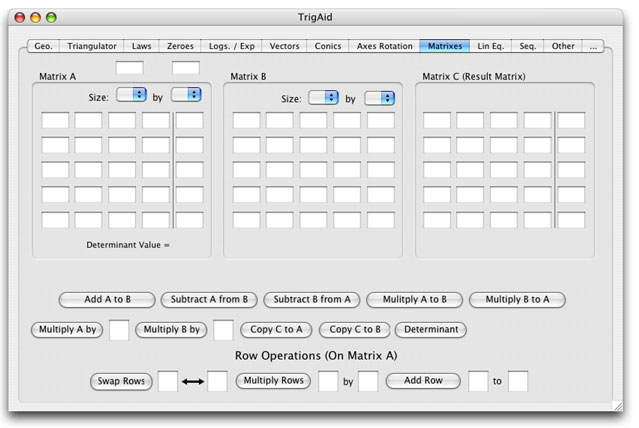Screenshot of the Matrix Tab

Conclusion

Don't waste valuable time searching through volumes of math books looking for the correct formula, or struggling with bulky, unintuitive calculators. Buy TrigAid NOW and leave the wasted time and frustration behind!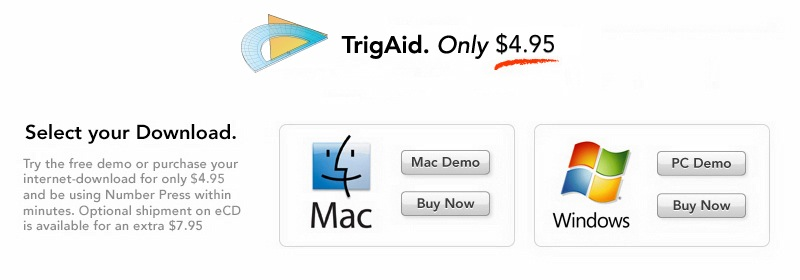a

 Macintosh Requirements PC Requirements Monitor resolution 1024x768 or higher REQUIRED Mac OS X Monitor resolution 1024x768 or higher REQUIRED Windows 2000-XP
 NOTE: If you have an older system than this, the software may still work. We encourage you to download the demo to try it. PDF and multiple-page PDF import require Mac OS X.